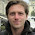## Friday, August 24, 2012

### Update on class structure, lattice constructors, plotting

Sorry that I haven't posted to this blog for a while. That doesn't mean that nothing happened in the meanwhile—on the contrary, I got quite a lot done in the last weeks of this Summer of Code.

First of all, I completely redesigned the class structure for lattices in Sage. Accounting for the discussion on sage-devel, a lattice in Sage is now a FreeQuadraticModule_ambient_pid with a given inner product matrix ("Gram matrix") and the "standard" basis [(1, 0, 0, ...), (0, 1, 0, ...), ...]. Additionally, a basis for the embedding of the lattice is stored. When constructing a lattice, either a Gram matrix or an embedded basis can be given, and the respective other property will be calculated (either using Cholesky decomposition or via the standard real inner product).

A lattice can also be constructed using a quadratic form or an order in an algebraic number field. The inner product matrix will be calculated accordingly.

There are only two lattice classes now: Lattice_with_basis is meant to be the base class for all lattices in Sage, while Lattice_ZZ represents lattices with base ring ZZ and integral Gram matrix. Storing the Gram matrix as well and not only the basis has the advantage that some lattices with floating-point basis can still be represented exactly, e.g., the embedding of ZZ[sqrt(2)] as a lattice in the sense of Minkowski's geometry of numbers. LLL reduction is primarily performed on the Gram matrix, and the embedded basis is transformed accordingly.

I also added new ways of creating lattices: random_lattice uses random_matrix to create a random basis of a given dimension and turns it into a ZZ-lattice. special_lattice can be used to create particular "special" named lattices such as the Leech lattice. The list of integrated named lattices is probably not complete, but easily extensible. Again, lattices can be specified using their basis or their Gram matrix.

Finally, 2-dimensional and 3-dimensional lattices can be plotted now. All combinations of basis vectors are plotted as points, and the extensive graphics capabilities of Sage allow to modify the plot further. For instance, the Voronoi cell can easily be added, too:

This graphics was constructed using the following code:

```L = special_lattice('SimpleCubic')
graphics = plot_lattice(L)
V = L.voronoi_cell()
graphics += V.plot()
graphics.show(viewer='tachyon')```

How do you like it?

1.Does your random_lattice/special_lattice command also interface with http://sagemath.org/doc/reference/sage/crypto/lattice.html ?

1.Thanks for your input! There is no direct interface (yet), but you can easily create a Lattice from crypto's gen_lattice by passing on the basis to Lattice:
L = Lattice(crypto.gen_lattice(...))
Do you think there should be a more direct connection?

2.Its a nice post thank you for sharing.
Best Oracle Training in Bangalore

3.Great Post. The information provided is of great use as I got to learn new things. Keep BloggingOracle Applications Training in Bangalore
.